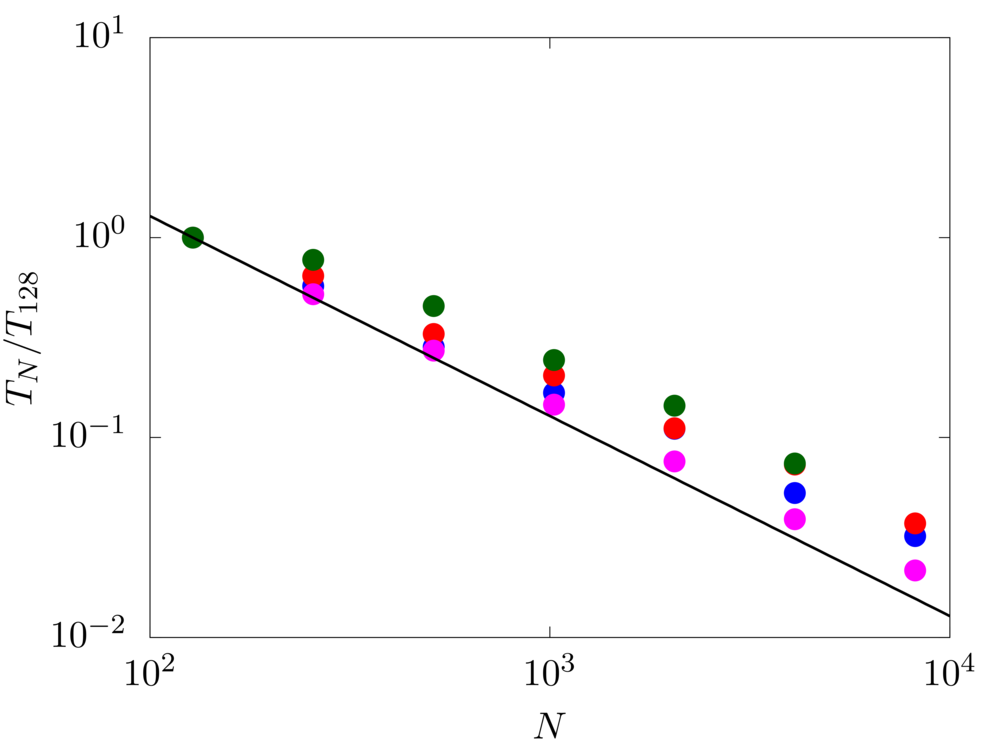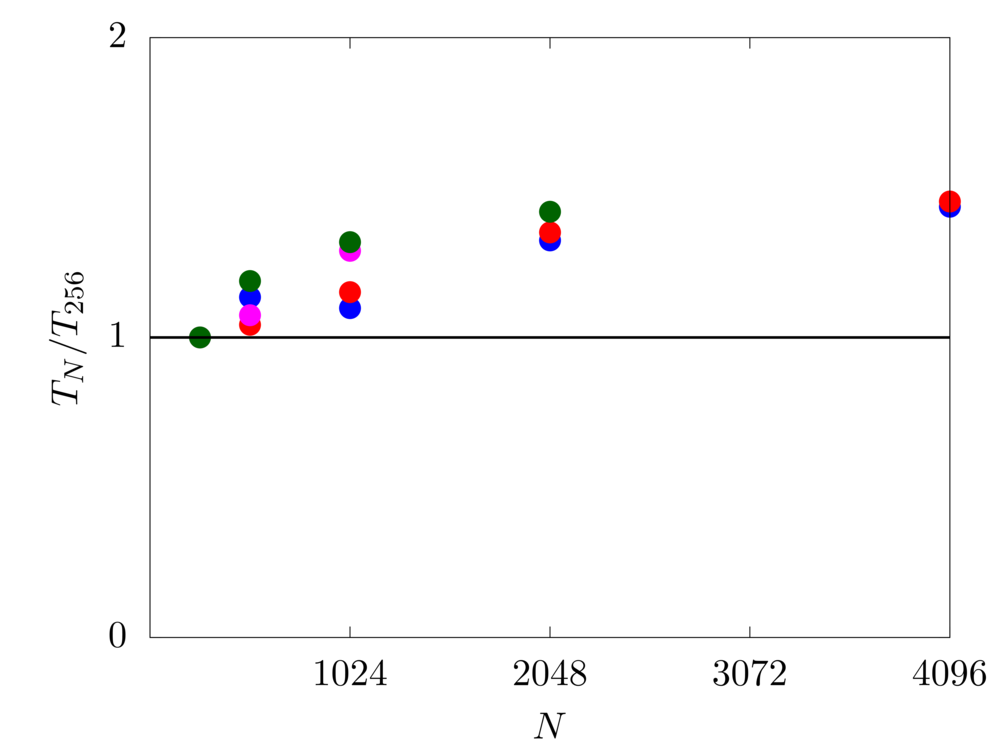# Code - Fujin - Scaling

We test the scaling performance of our solver. In particular, we consider the time evolution of a Taylor-Green vortex in a three-periodic domain of size $$2\pi L$$. The velocity field is initialized as

$$u = U \sin \left( x/L \right) \cos \left( y/L \right) \cos \left( z/L \right),$$

$$v = -U \cos \left( x/L \right) \sin \left( y/L \right) \cos \left( z/L \right),$$

$$w=0.$$

The Reynolds number $$Re = \rho U L /\mu$$ is fixed equal to $$1600$$.Strong scaling of the numerical method in a domain with 1024 grid cells per side. Blue: Beskow - KTH (Sweden) Red: Oakbridge-CX - UTokyo (Japan) Magenta: Oakforest-PACS - JCAHPC (Japan) Green: Deigo - OIST (Japan)

The strong scaling test evaluates the speedup for a fixed problem size with respect to the number of processors. For this test, we consider a numerical domain discretised with $$1024$$ grid cell per side. The code shows very good scaling performance up to about $$10^4$$cores, with an almost linear decay of the computational time with the number of cores used.Weak scaling of the numerical method in a domain with 8M grid cells per sub-domain. Blue: Beskow - KTH (Sweden) Red: Oakbridge-CX - UTokyo (Japan) Magenta: Oakforest-PACS - JCAHPC (Japan) Green: Deigo - OIST (Japan)

The weak scaling test evaluates the speedup for a scaled problem size with respect to the number of processors. For this test, we consider a numerical domain discretised with around $$8M$$ grid cells per computational sub-domain. The code shows a good scaling with only a small decay of performance from $$N=256$$ to $$4096$$ cores, suggesting that strong scaling timings can scale up to much larger problem sizes.